# Intermediate Maths Solutions for The Straight Line Exercise 3(a)

The Straight Line Exercise 3(a) Maths 1b textbook Chapter 3 Inter Solutions are given.

All problems with answers are given. These are very simple to understand.

Observe the example problems and solutions in the textbook.

Observe the given solutions and try them in your own method.

You can also see

SSC Maths text book Solutions class 10

Inter Maths 1A Solutions textbook

Inter Maths 1B textbook solutions

Inter Maths 1IA text book solutions

Inter Maths IIB text book solutions

The Straight Line

Exercise 3(a)

Exercise 3(b)

Exercise 3(c)

Exercise 3(d)

Exercise 3(e)

M

## Inter 1b Chapter 3 The Straight Line Exercise 3(a) Solutions

Chapter 3 The Straight line Inter 1b

Exercise 3(a) Solutions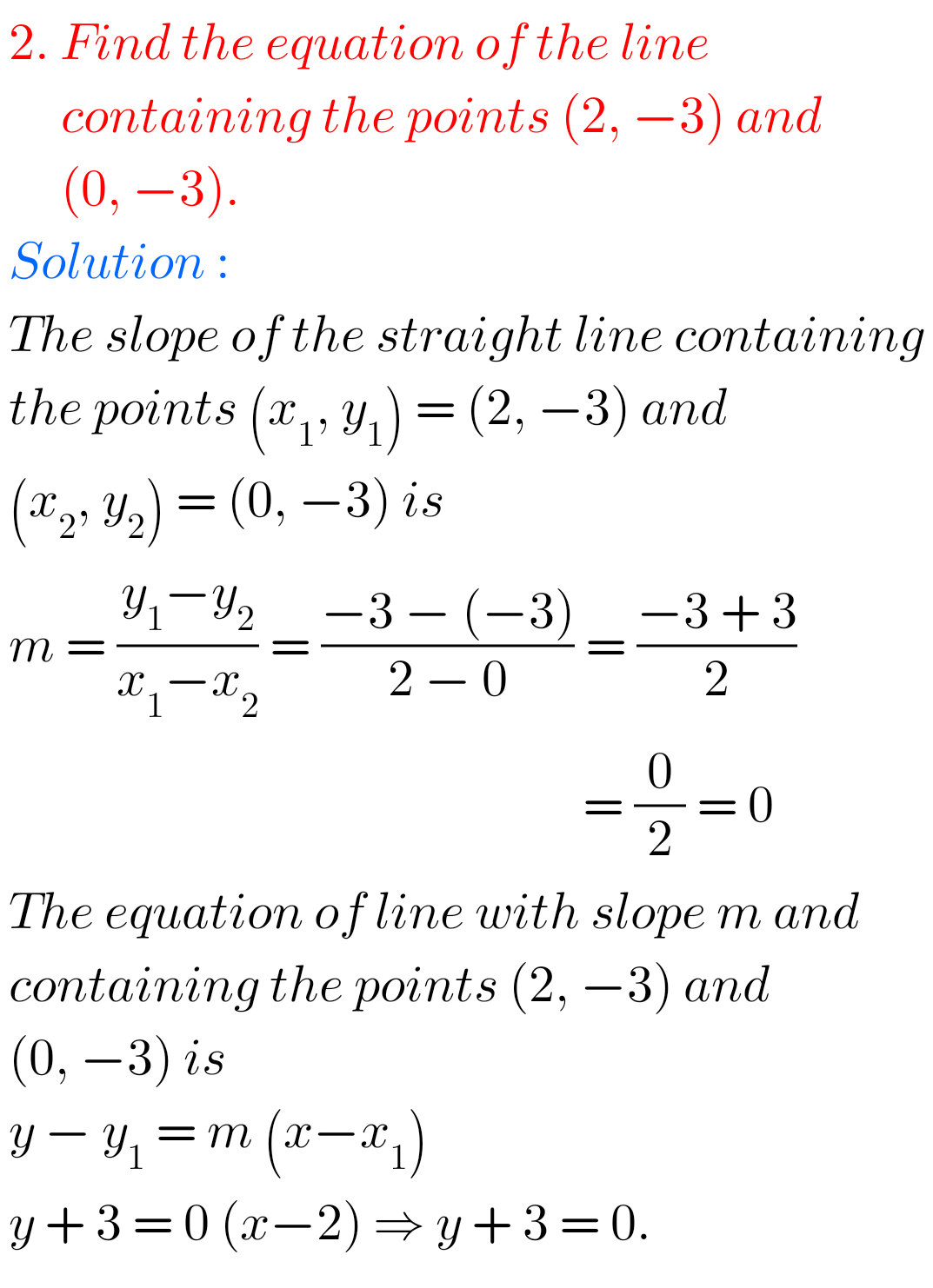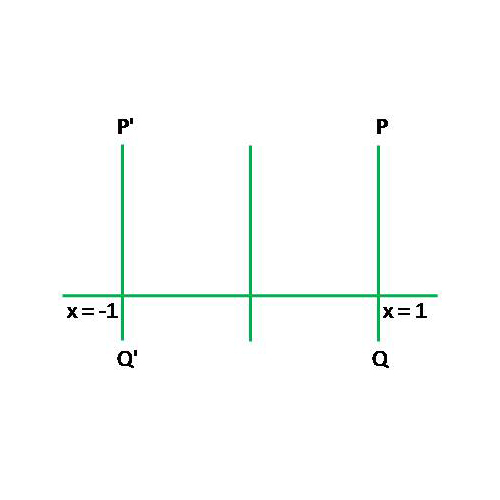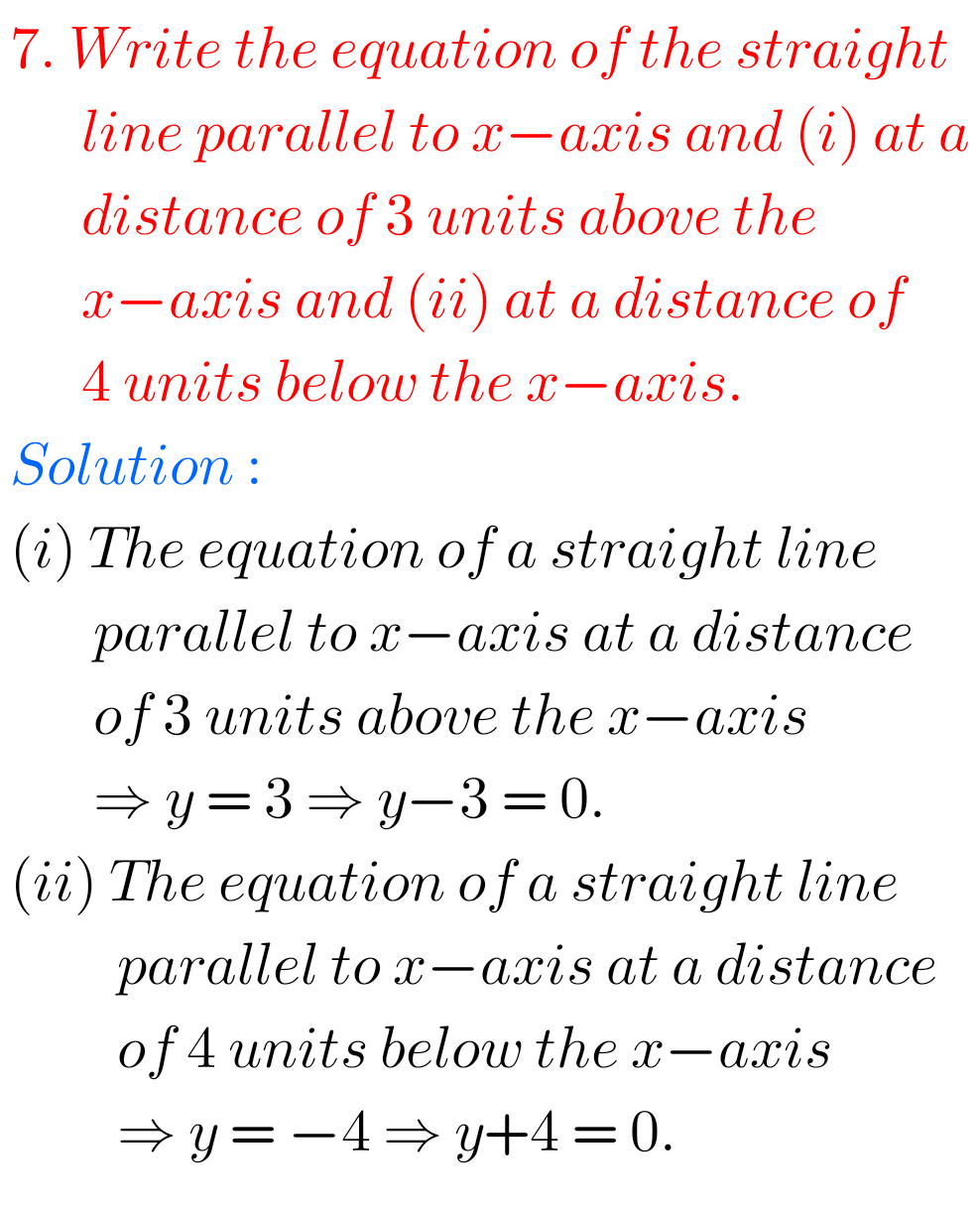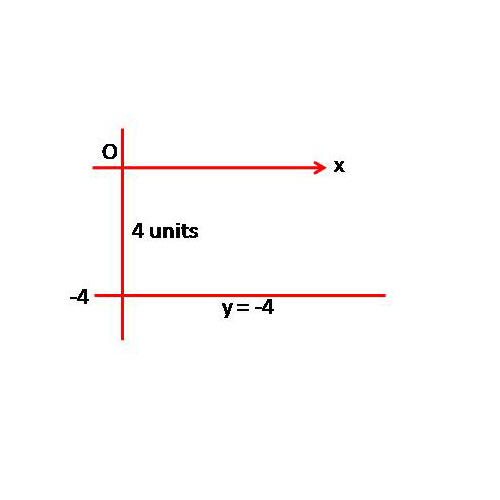##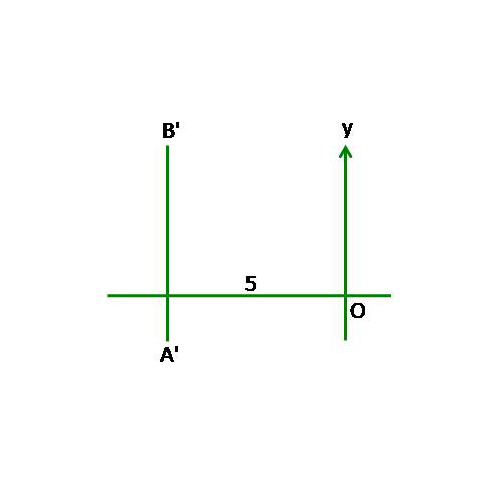## The Straight Line Exercise 3(a) Solutions Inter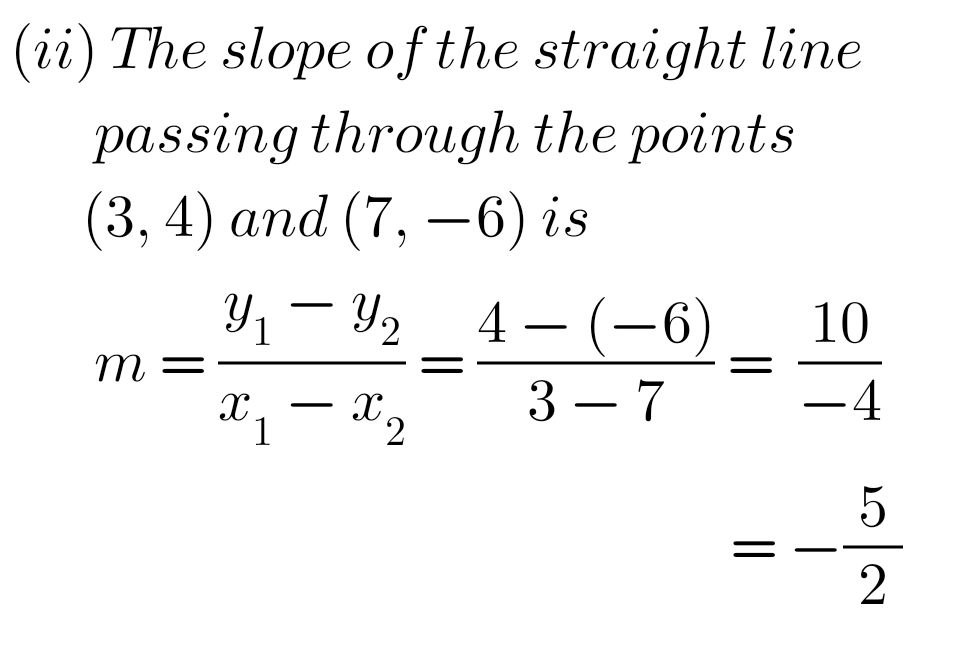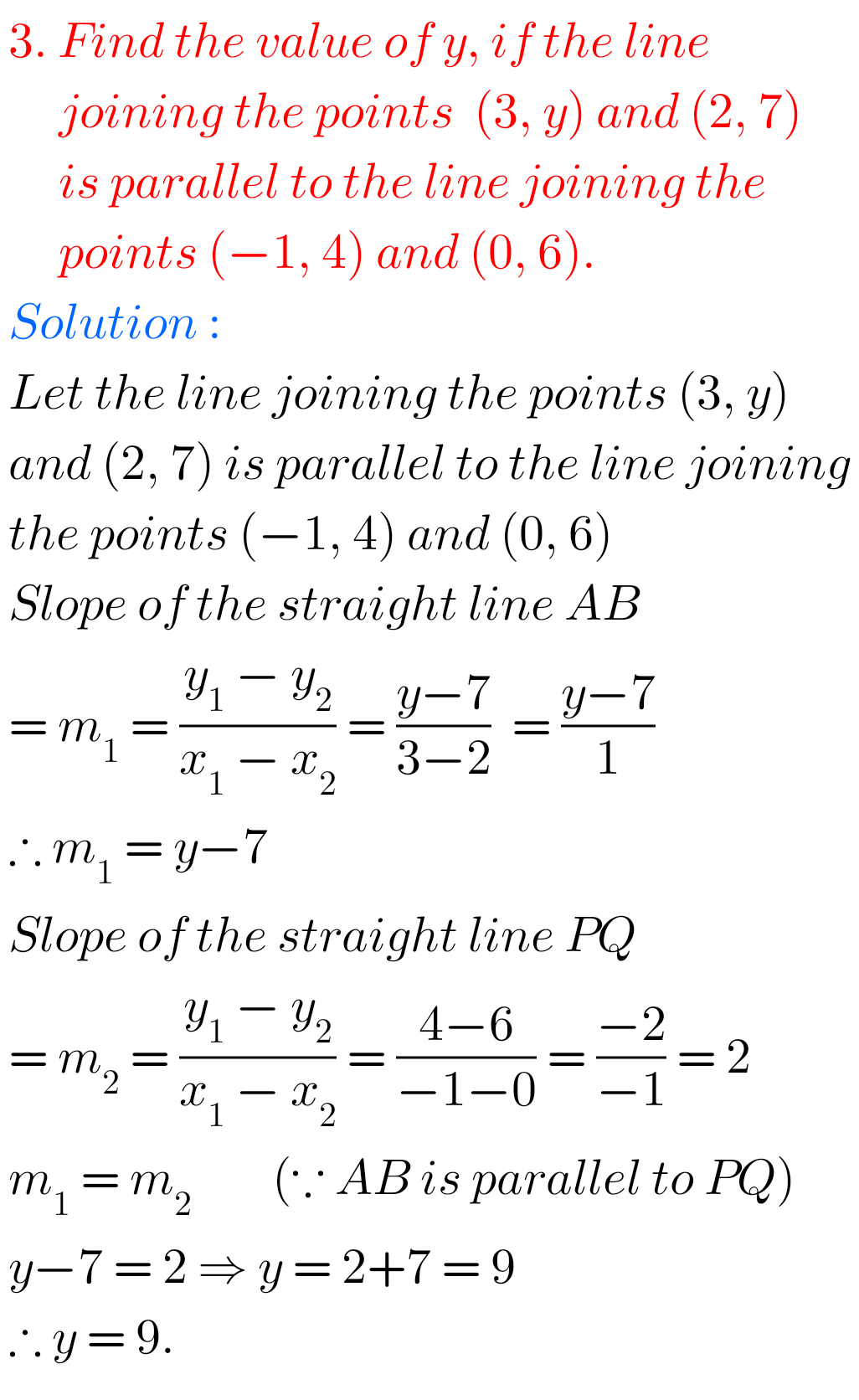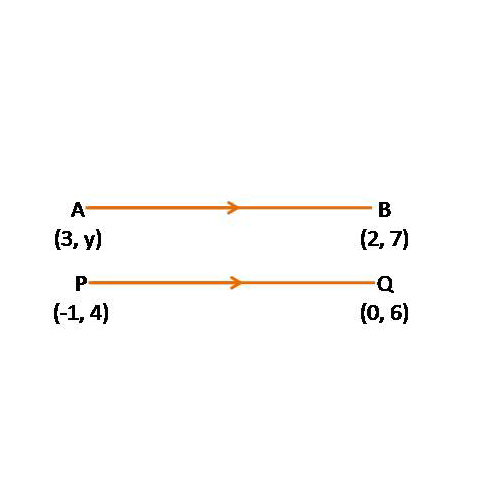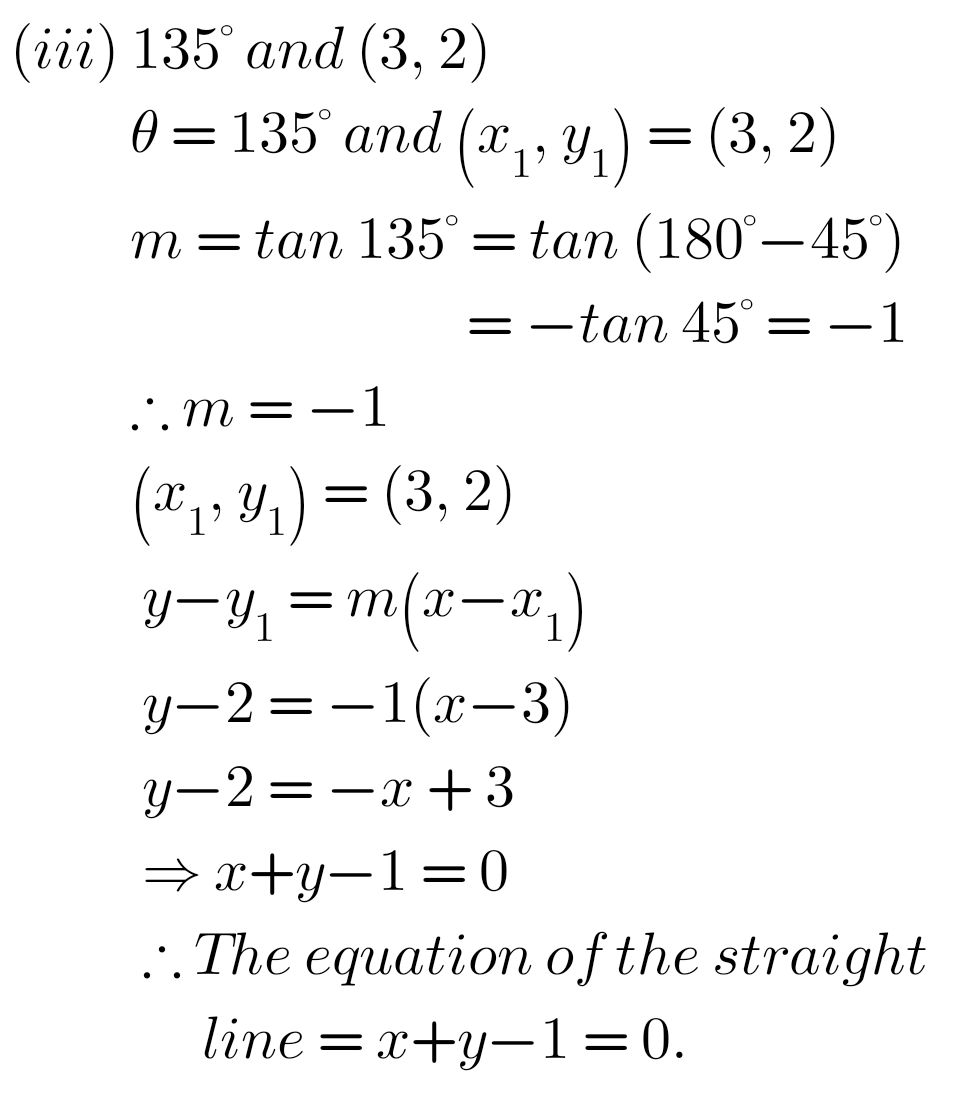## Solutions for Exercise 3(a) The Straight Line Inter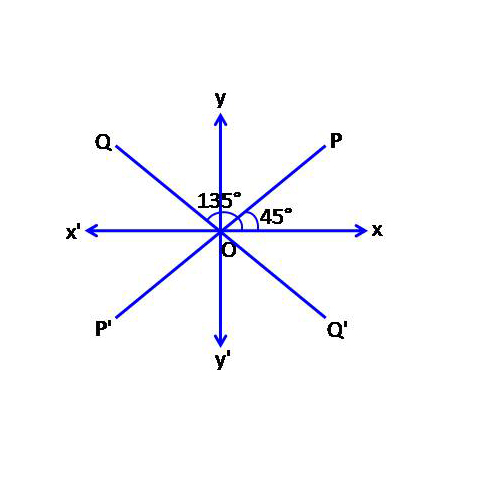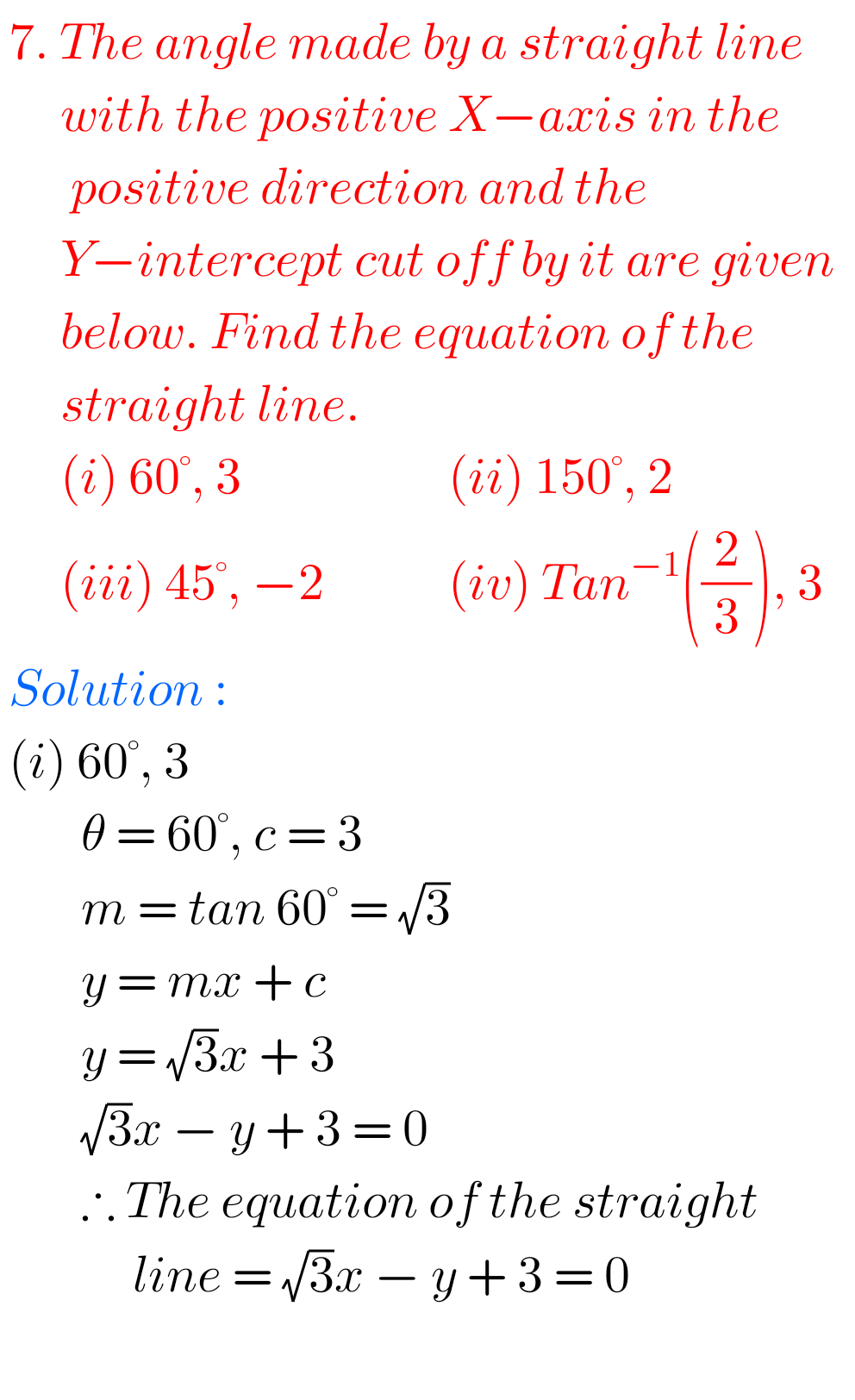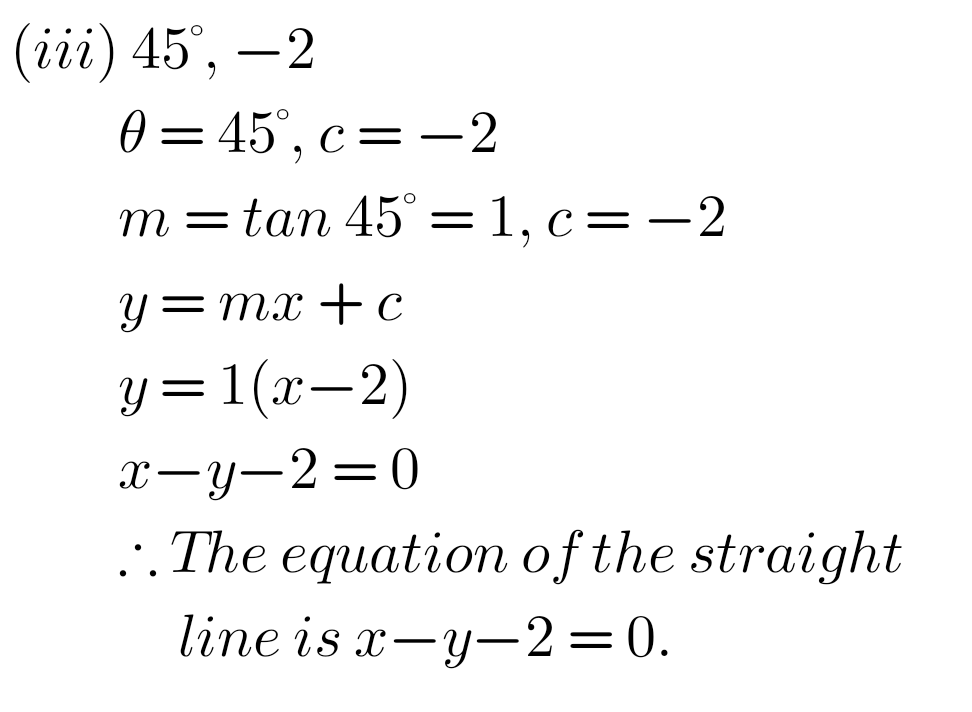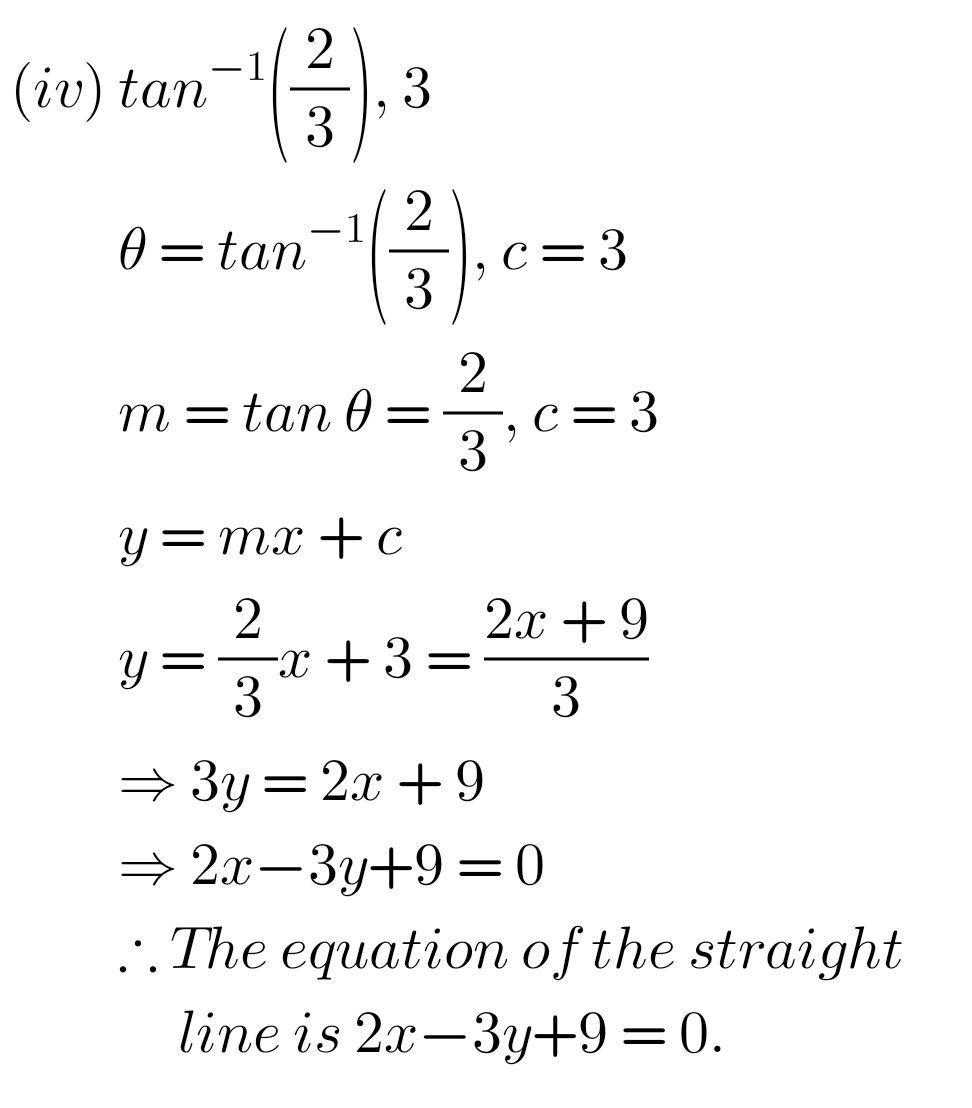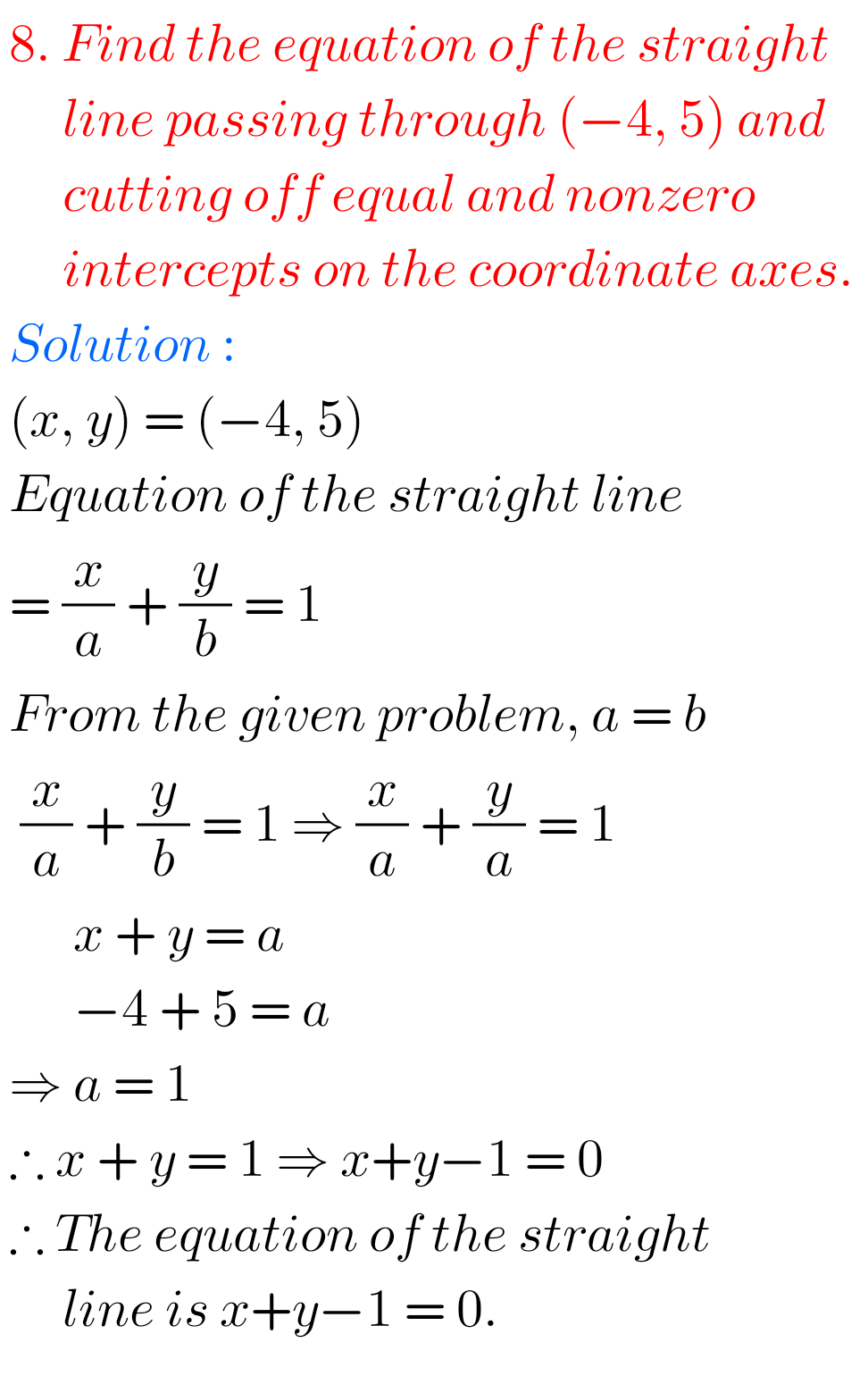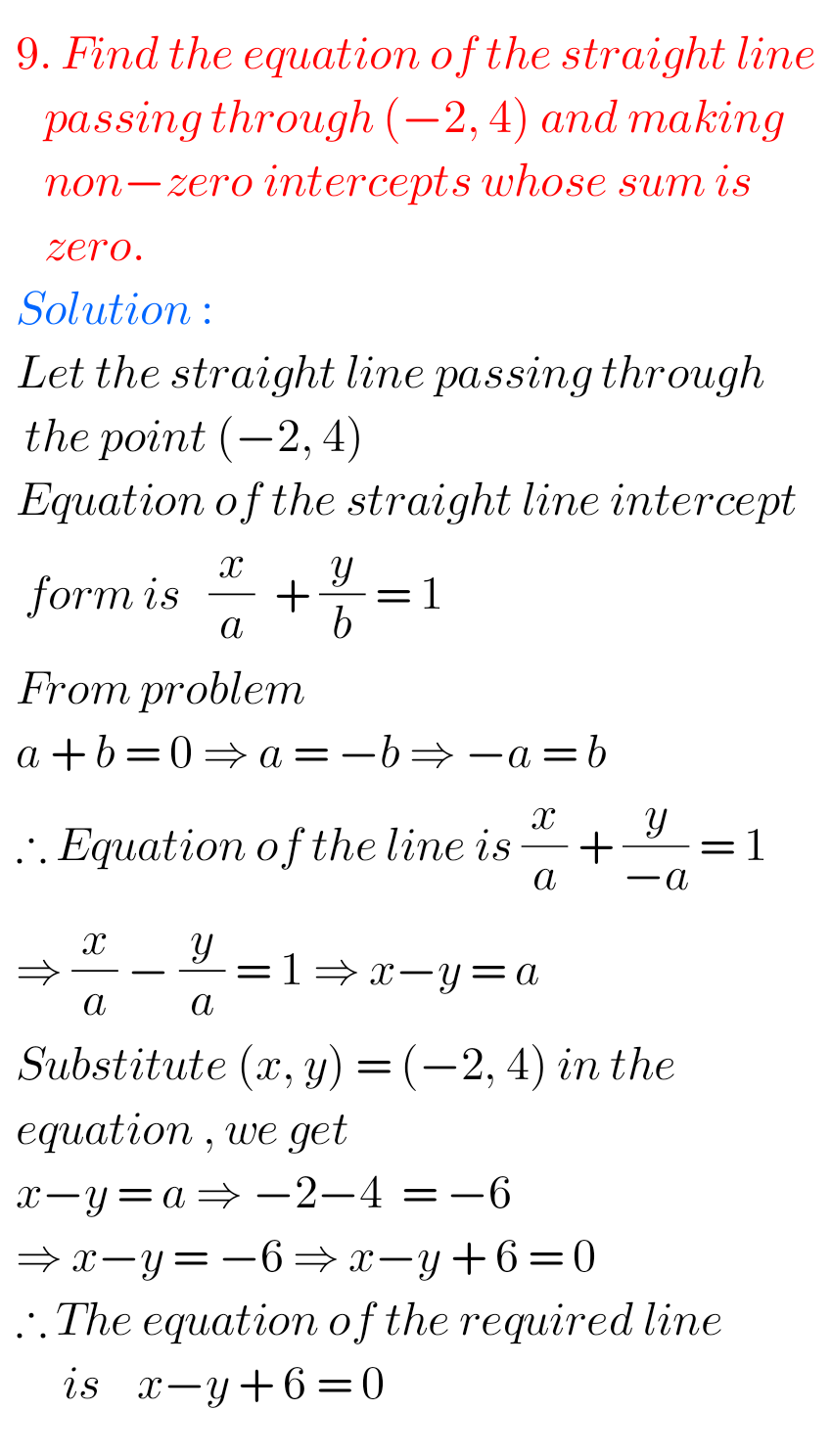M

### Maths Solutions for Exercise 3(a) The Straight Line Inter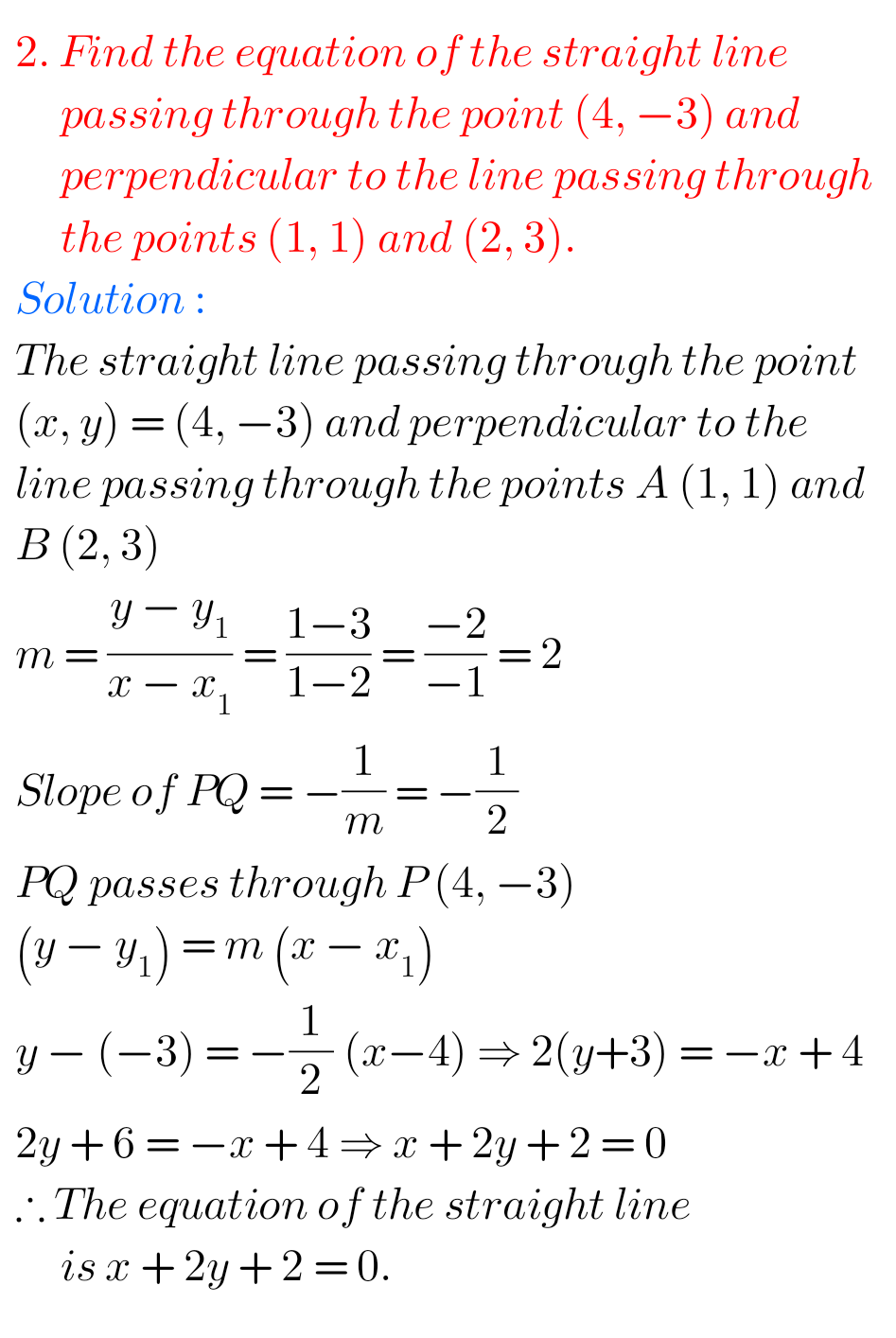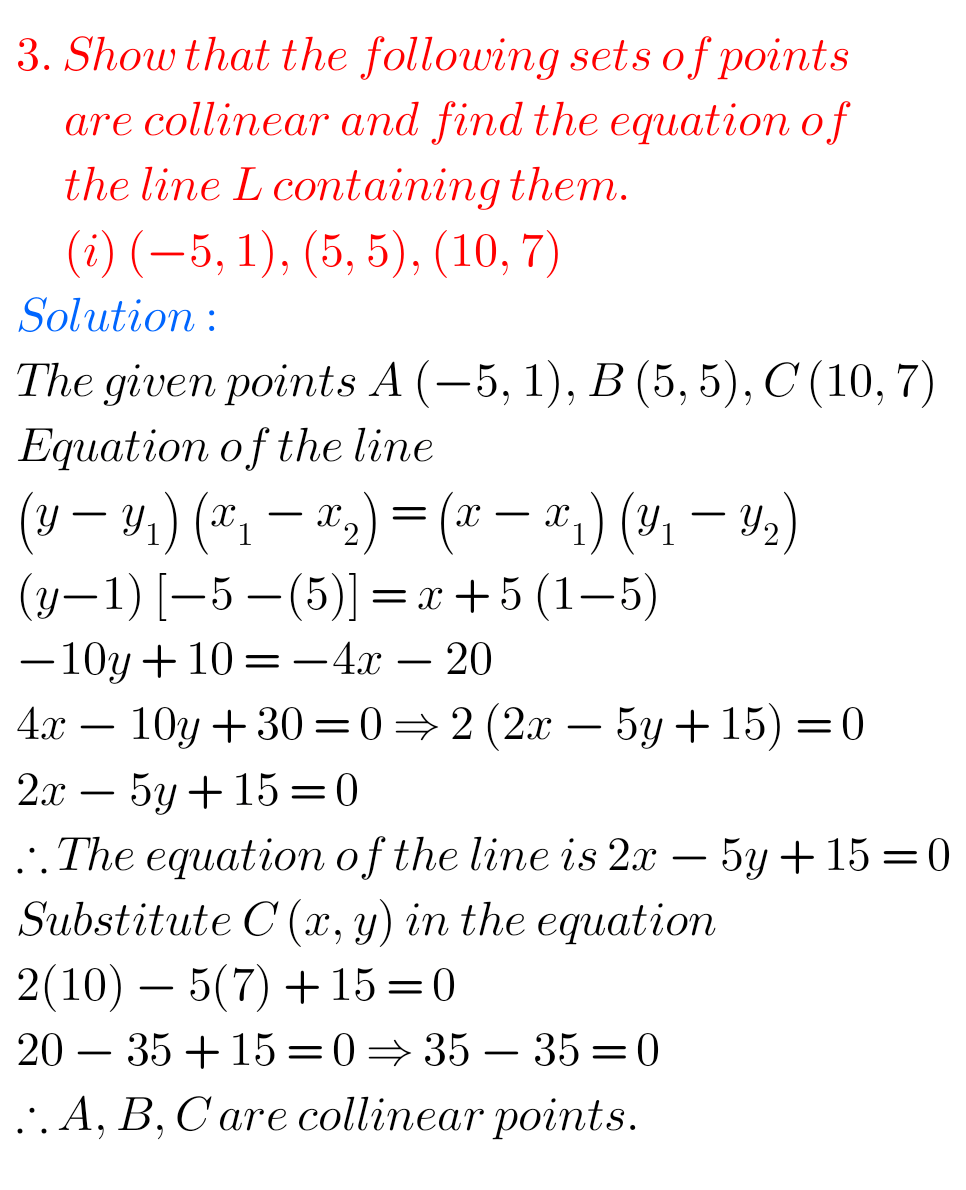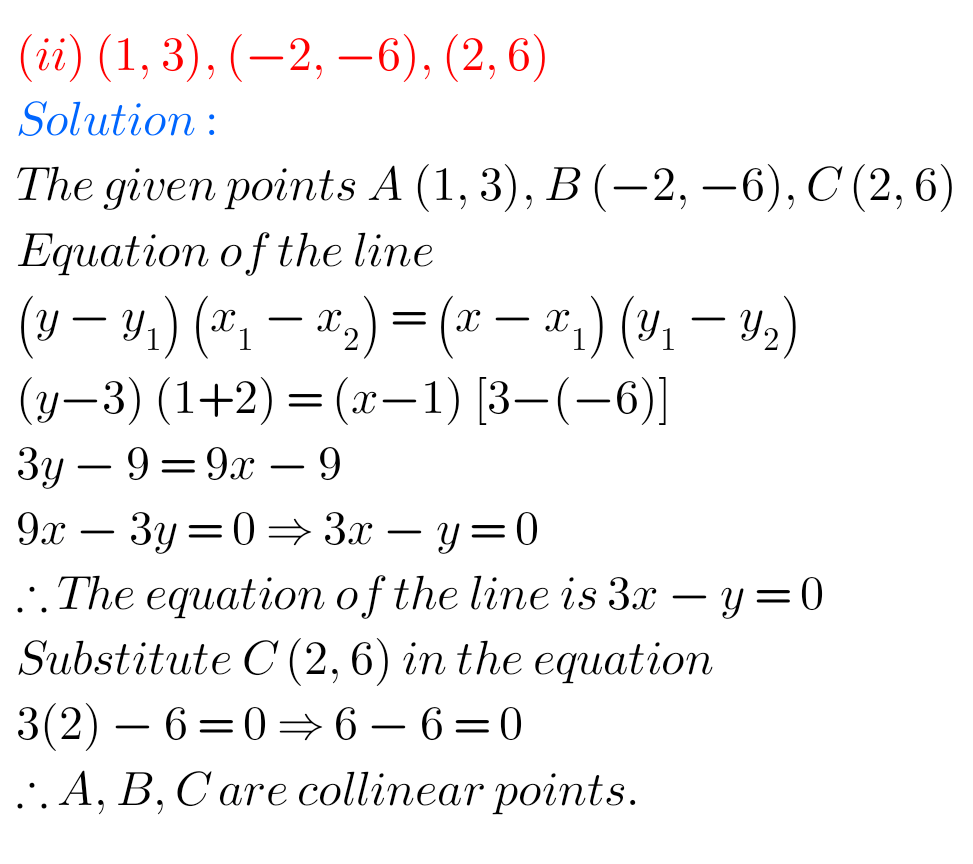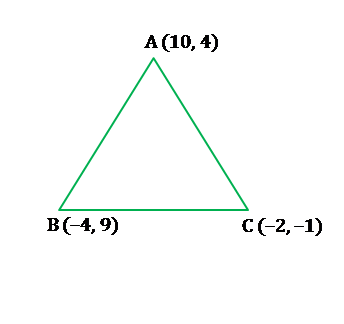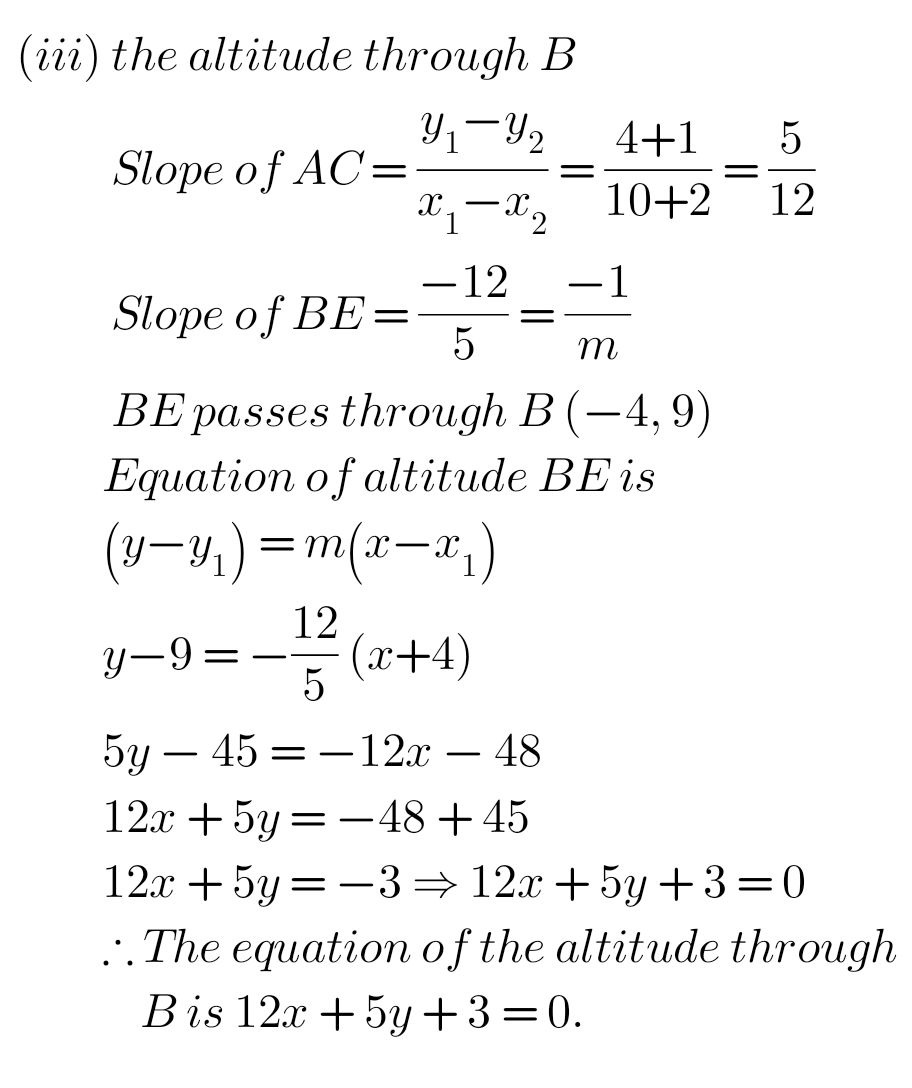Note : Observe the solutions and try them in your own method.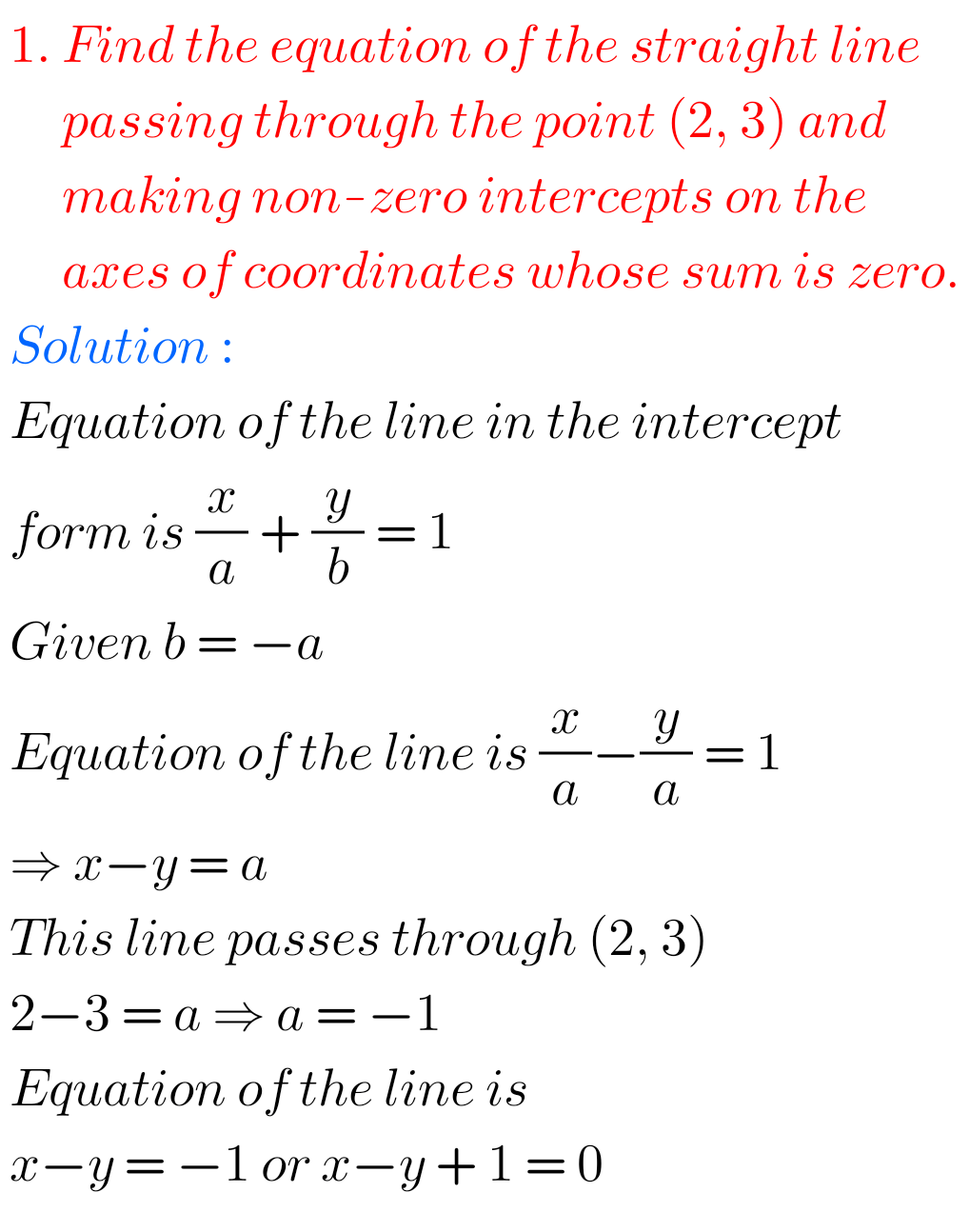Ncert maths class 6 chapter 14 solutions

Nios maths 311 book 1 Sets 1.1 solutions